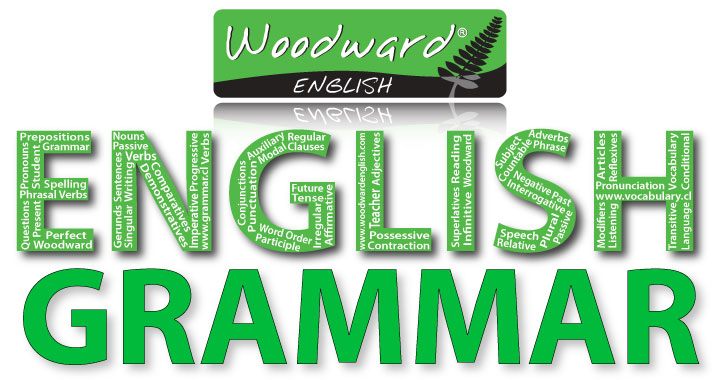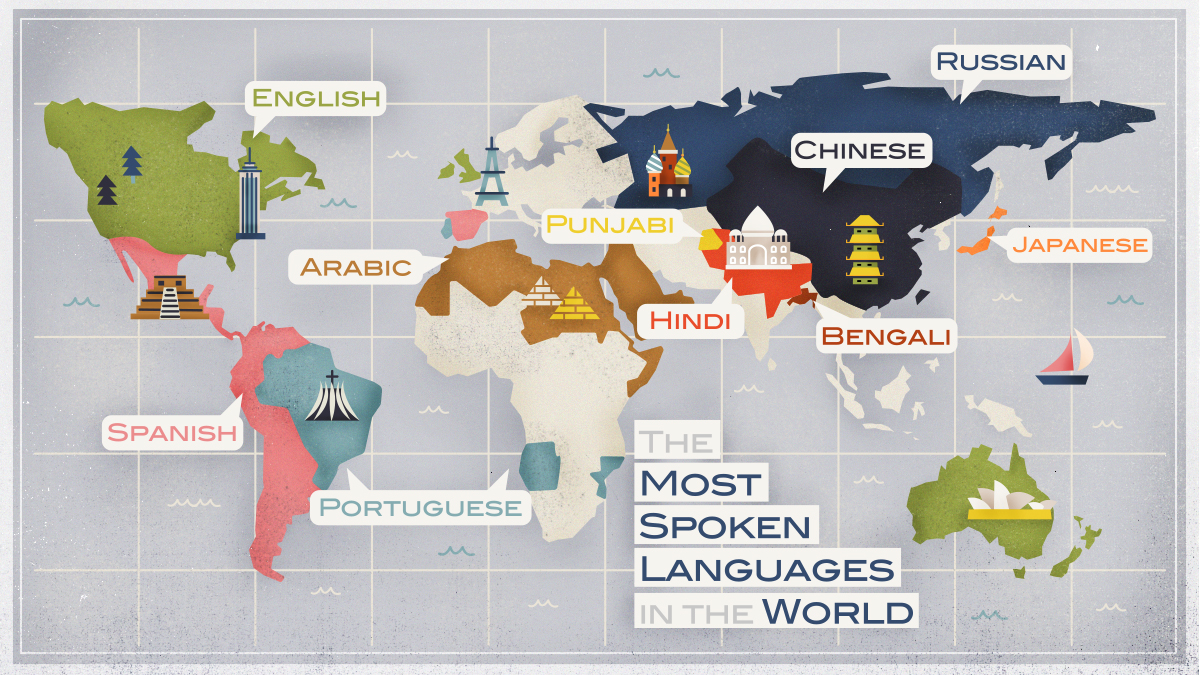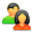TweetONLINE
4
Partilhe esta Página

Total de visitas: 262556
Top 70+ Most Popular Contractions

Top 70+ Most Popular Contractions in English with Examples

Below are the contractions definition and list of commonly used contractions in English.

What is a Contraction?

Contractions definition: We use contractions (I’m, we’re) in everyday speech and informal writing. Contractions, which are sometimes called “short forms”, commonly combine a pronoun or noun and a verb, or a verb and not, in a shorter form. Contractions are usually not appropriate in formal writing.

Examples of Contractions

• Yes, please, I’d love a coffee.
• They’ve been asking a lot of questions.
• He’s my brother.
• I’ll be with you in spirit.
• She’s been invited to a party.
• You’re never too old to learn.
• I’d a big argument with my mother this morning.
• She’ll appear in court tomorrow.
• There’s a distinct improvement in your English.
• Who’s the woman in the black hat?

List of Contractions in English

Would

• I would = I’d
• You would = You’d
• He would = He’d
• She would = She’d
• It would = It’d
• We would = We’d
• They would = They’d
• That would = That’d
• These would = These’d
• There would = There’d
• Who would = Who’d
• What would = What’d
• Where would = Where’d
• When would = When’d
• Why would = Why’d
• How would = How’d

Be

• You are = You’re
• He is = He’s
• She is = She’s
• It is = It’s
• We are = We’re
• They are = They’re
• That is = That’s
• These are = These’re
• There is = There’s
• Who is = Who’s
• What are = What’re
• Where is = Where’s
• When is = When’s
• Why is = Why’s
• How are = How’re

Have/ Has

• I have = I’ve
• You have = You’ve
• He has = He’s
• She has = She’s
• It has = It’s
• We have = We’ve
• They have = They’ve
• That has = That’s
• These have = These’ve
• There has = There’s
• Who has = Who’s
• What have = What’ve
• Where has = Where’s
• Why has = Why’s
• How have = How’ve

• I had = I’d
• You had = You’d
• He had = He’d
• She had = She’d
• It had = It’d
• We had = We’d
• They had = They’d
• That had = That’d
• These had = These’d
• There had = There’d
• Who had = Who’d
• What had = What’d
• Where had = Where’d
• Why had = Why’d
• How had = How’d

Will

• I will = I’ll
• You will = You’ll
• He will = He’ll
• She will = She’ll
• It will = It’ll
• We will = We’ll
• They will = They’ll
• That will = That’ll
• These will = These’ll
• There will = There’ll
• Who will = Who’ll
• What will = What’ll
• Where will = Where’ll
• When will = When’ll
• Why will = Why’ll
• How will = How’ll

Most Popular Contractions | Picture